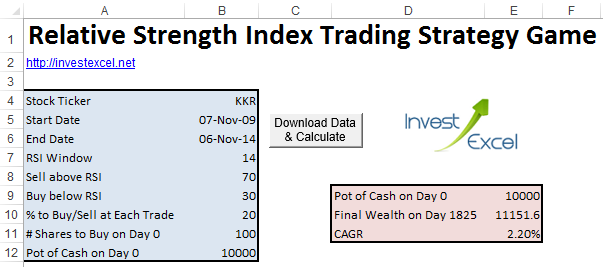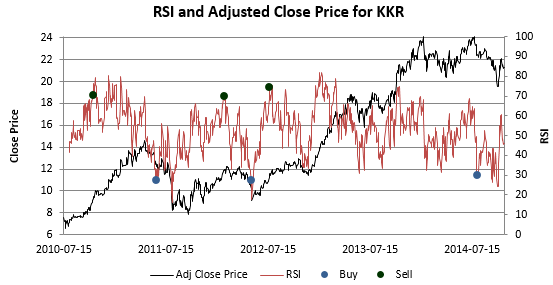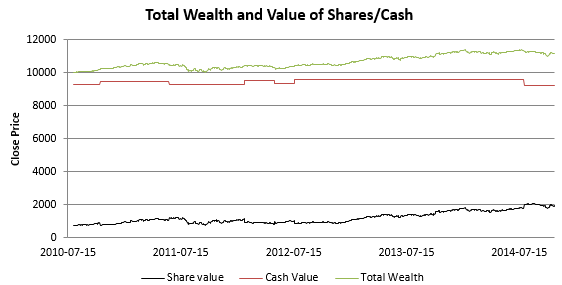Backtest a simple RSI trading strategy with this web-connected spreadsheet – play a fantasy stock trading game!

The spreadsheet downloads historic prices for your chosen ticker, and some VBA triggers buy or sell points when the relative strength index (RSI) rises above or falls below user-defined values.

The trading logic is not sophisticated or complex (it’s described in greater detail below).

But you can use similar principles to develop and backtest enhanced strategies. For example, you could code a scheme that uses several indicators (such as ATR or the stochastic oscillator) to confirm trends before triggering buy/sell points.

• it’s not a realistic trading strategy
• no transaction costs or other factors are included
• the VBA demonstrates how you might code a simple backtesting algorithm – feel free to enhance it, tear it apart or just plain geek out

But most importantly, it’s a game – change parameters, try new stock and have fun! For example, the spreadsheet calculates the compound annual growth rate of your investment pot; try to get this number as high as possible.• a stock ticker, a start date, and an end date
• an RSI window
• the value of RSI above which you want to sell a fraction of your stock
• the value of RSI below which you want to sell a fraction of your stock
• the fraction of shares to buy or sell at each trade
• the amount of money you have on day 0
• the number of shares to buy on day 0

After you click a button, some VBA starts ticking away behind the scenes and

• downloads historical stock prices between the start date and end date from Yahoo
• calculates the RSI for each day between the start and end date (removing, of course, the initial RSI window)
• on Day 0 (that’s the day before you start trading) buys a number of shares with your pot of cash
• from Day 1 onwards,sells a defined fraction of shares if RSI rises above a pre-defined value, or buys a fraction of shares if RSI falls below a pre-defined value
• calculates the compound annual growth rate, taking into account the value of the original pot of cash, the final value of cash and shares, and the number of days spent trading.

Bear in mind that if RSI triggers a sell, the logic has to trigger a buy before a sell can be triggered again (and vice-versa). That is, you can’t have two sell triggers or two buy triggers in a row.

You also get a plot of the close price, RSI and the buy/sell pointsYou also get a plot of your total fantasy wealth grows over time.The buy/sell points are calculated with the following VBA – following the logic is easy

``` For i = RSIWindow + 2 To numRows If Sheets("Data").Range("N" & i) > sellAboveRSI And state = 0 Then Sheets("Data").Range("O" & i) = "Sell" '# Shares Sheets("Data").Range("P" & i).Formula = "=-int(-(1 - pxBuySell/100) * P" & i - 1 & ")" 'Cash value Sheets("Data").Range("R" & i).Formula = "=R" & i - 1 & " -int(- pxBuySell/100 * P" & i - 1 & ")* G" & i state = 1 ElseIf _ Sheets("Data").Range("N" & i) < buyBelowRSI _ And Sheets("Data").Range("R" & i - 1) > pxBuySell / 100 * Sheets("Data").Range("P" & i - 1) * Sheets("Data").Range("G" & i) _ And state = 1 Then Sheets("Data").Range("O" & i) = "Buy" '# Shares Sheets("Data").Range("P" & i).Formula = "=-int(-(1 + pxBuySell/100) * P" & i - 1 & ")" 'Cash value Sheets("Data").Range("R" & i).Formula = "=R" & i - 1 & " - pxBuySell/100 * P" & i - 1 & "* G" & i state = 0 Else Sheets("Data").Range("P" & i).Formula = "=P" & i - 1 Sheets("Data").Range("R" & i).Formula = "=R" & i - 1 Sheets("Data").Range("O" & i) = "Hold"   End If 'Share Value Sheets("Data").Range("Q" & i).Formula = "=G" & i & " * P" & i 'Total Value Sheets("Data").Range("S" & i).Formula = "=Q" & i & " + R" & i 'Buy/Sell Points Sheets("Data").Range("T" & i).Formula = "=if(O" & i & "=""Buy"",N" & i & ",-200)" Sheets("Data").Range("U" & i).Formula = "=if(O" & i & "=""Sell"",N" & i & ",-200)" Next```

View the rest of the VBA in Excel (there’s lots there to learn from)

If you’re suitably caffeinated, you could enhance the VBA to employ other indicators to confirm trading points; for example, you could trigger selling points only if RSI rises above 70 and MACD falls below its signal line.### 8 thoughts on “RSI Trading Strategy Game”

1. This calculator implies that the closer you set the buy/sell indicators to 50, the higher the final wealth. This can be exemplified by entering the following parameters. Is it possible that this is incorect?

Stock Ticker VTI
Start Date 16-Nov-09
End Date 15-Nov-14
RSI Window 14
Sell above RSI 50.1
# Shares to Buy on Day 0 17
Pot of Cash on Day 0 1000

2. In Excel for Mac, the following statement in GetData produces a compile error:

For Each C In ThisWorkbook.Connections
C.Delete

• Does the VBA work on a Mac if you comment out those lines?

I’ve tested the spreadsheet on Excel 2010 and 2013 on Windows 8 and it’s fine

• I deleted out those lines and it seemed to run OK. Thanks.

3. RSI does not correct for splits.
Price does.